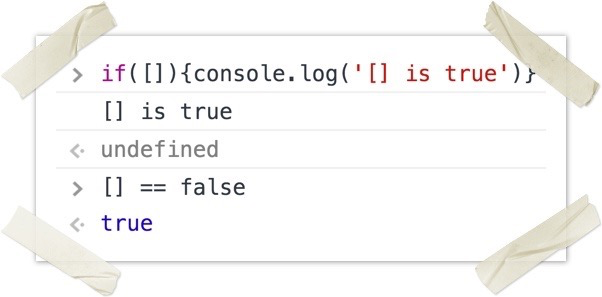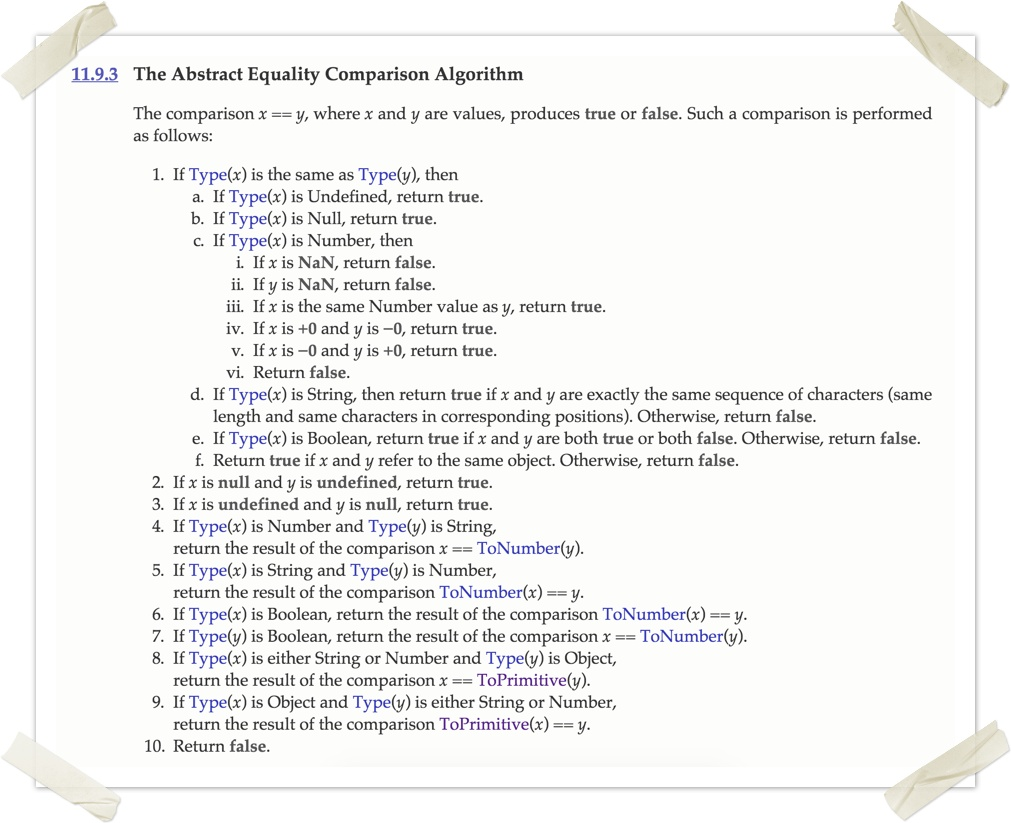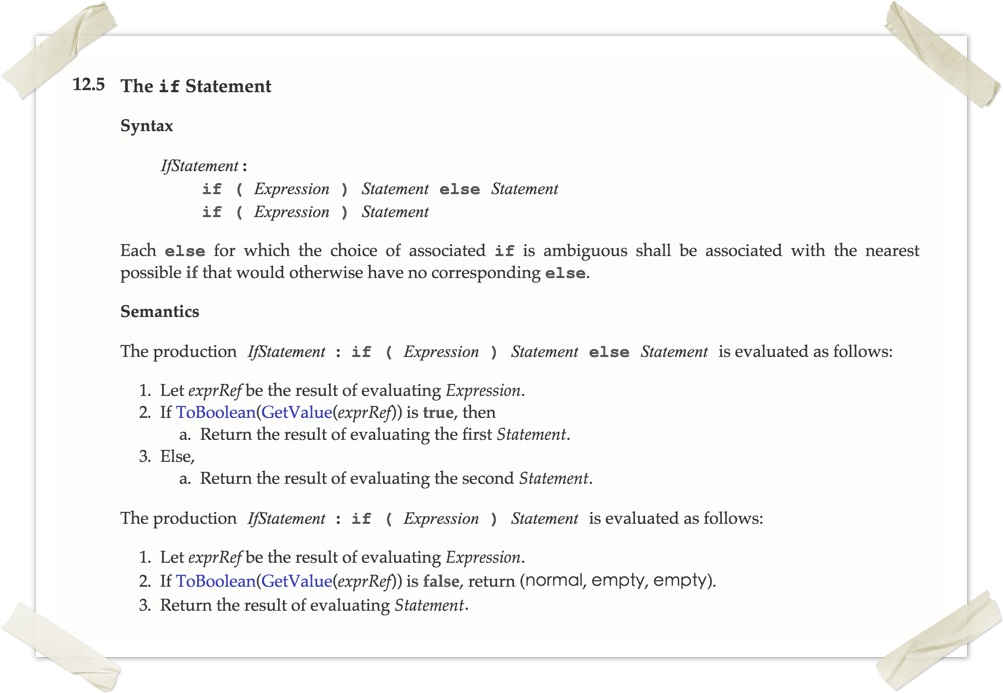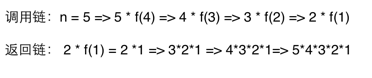### JavaScript客户端脚本语言

Javascript 是一种由Netscape的LiveScript发展而来的原型化继承的基于对象的动态类型的区分大小写的客户端脚本语言，主要目的是为了解决服务器端语言，比如Perl，遗留的速度问题，为客户提供更流畅的浏览效果。

1、使用 `typeof bar === "object"` 判断 `bar` 是不是一个对象有神马潜在的弊端？如何避免这种弊端？

``````let obj = {};
let arr = [];

console.log(typeof obj === 'object');  //true
console.log(typeof arr === 'object');  //true
console.log(typeof null === 'object');  //true``````

``````let obj = {};
let arr = [];

console.log(Object.prototype.toString.call(obj));  //[object Object]
console.log(Object.prototype.toString.call(arr));  //[object Array]
console.log(Object.prototype.toString.call(null));  //[object Null]```````[] === false` 是返回 `false` 的。

2、下面的代码会在 console 输出神马？为什么？

``````(function(){
var a = b = 3;
})();

console.log("a defined? " + (typeof a !== 'undefined'));
console.log("b defined? " + (typeof b !== 'undefined'));``````

``````console.log(b); //3
console,log(typeof a); //undefined``````

``````b = 3;
var a = b;``````

3、下面的代码会在 console 输出神马？为什么？

``````var myObject = {
foo: "bar",
func: function() {
var self = this;
console.log("outer func:  this.foo = " + this.foo);
console.log("outer func:  self.foo = " + self.foo);
(function() {
console.log("inner func:  this.foo = " + this.foo);
console.log("inner func:  self.foo = " + self.foo);
}());
}
};
myObject.func();``````

``````(function(test) {
console.log("inner func:  this.foo = " + test.foo);  //'bar'
console.log("inner func:  self.foo = " + self.foo);
}(self));``````

4、将 JavaScript 代码包含在一个函数块中有神马意思呢？为什么要这么做？

IIFE 有两个比较经典的使用场景，一是类似于在循环中定时输出数据项，二是类似于 JQuery/Node 的插件和模块开发。

``````for(var i = 0; i < 5; i++) {
setTimeout(function() {
console.log(i);
}, 1000);
}``````

``````for(var i = 0; i < 5; i++) {
(function(i) {
setTimeout(function() {
console.log(i);
}, 1000);
})(i)
}``````

``````(function(\$) {
//代码
} )(jQuery);``````

5、在严格模式('use strict')下进行 JavaScript 开发有神马好处？

• 消除Javascript语法的一些不合理、不严谨之处，减少一些怪异行为;

• 消除代码运行的一些不安全之处，保证代码运行的安全；

• 提高编译器效率，增加运行速度；

• 为未来新版本的Javascript做好铺垫。

6、下面两个函数的返回值是一样的吗？为什么？

``````function foo1()
{
return {
bar: "hello"
};
}

function foo2()
{
return
{
bar: "hello"
};
}``````

``````var test = 1 +
2
console.log(test);  //3``````

``````function foo2()
{
return;
{
bar: "hello"
};
}``````

7、神马是 NaN，它的类型是神马？怎么测试一个值是否等于 NaN?

`NaN` 是 Not a Number 的缩写，JavaScript 的一种特殊数值，其类型是 Number，可以通过 `isNaN(param)` 来判断一个值是否是 `NaN`

``````console.log(isNaN(NaN)); //true
console.log(isNaN(23)); //false
console.log(isNaN('ds')); //true
console.log(isNaN('32131sdasd')); //true
console.log(NaN === NaN); //false
console.log(NaN === undefined); //false
console.log(undefined === undefined); //false
console.log(typeof NaN); //number
console.log(Object.prototype.toString.call(NaN)); //[object Number]``````

ES6 中，`isNaN()` 成为了 Number 的静态方法：`Number.isNaN()`.

8、解释一下下面代码的输出

``````console.log(0.1 + 0.2);   //0.30000000000000004
console.log(0.1 + 0.2 == 0.3);  //false``````

JavaScript 中的 number 类型就是浮点型，JavaScript 中的浮点数采用IEEE-754 格式的规定，这是一种二进制表示法，可以精确地表示分数，比如1/2，1/8，1/1024，每个浮点数占64位。但是，二进制浮点数表示法并不能精确的表示类似0.1这样 的简单的数字，会有舍入误差。

``````0.1 => 0.0001 1001 1001 1001…（无限循环）
0.2 => 0.0011 0011 0011 0011…（无限循环）``````

``````function add(num1, num2){
let r1, r2, m;
r1 = (''+num1).split('.').length;
r2 = (''+num2).split('.').length;

m = Math.pow(10,Math.max(r1,r2));
return (num1 * m + num2 * m) / m;
}

``````function add(x, y) {
return x.toPrecision() + y.toPrecision()
}

9、实现函数 `isInteger(x)` 来判断 x 是否是整数

``````function isInteger(x) {
return parseInt(x, 10) === x;
}``````

ES6 对数值进行了扩展，提供了静态方法 `isInteger()` 来判断参数是否是整数：

``````Number.isInteger(25) // true
Number.isInteger(25.0) // true
Number.isInteger(25.1) // false
Number.isInteger("15") // false
Number.isInteger(true) // false``````

JavaScript能够准确表示的整数范围在 `-2^53``2^53` 之间（不含两个端点），超过这个范围，无法精确表示这个值。ES6 引入了`Number.MAX_SAFE_INTEGER``Number.MIN_SAFE_INTEGER`这两个常量，用来表示这个范围的上下限，并提供了 `Number.isSafeInteger()` 来判断整数是否是安全型整数。

10、在下面的代码中，数字 1-4 会以什么顺序输出？为什么会这样输出？

``````(function() {
console.log(1);
setTimeout(function(){console.log(2)}, 1000);
setTimeout(function(){console.log(3)}, 0);
console.log(4);
})();``````

11、写一个少于 80 字符的函数，判断一个字符串是不是回文字符串

``````function isPalindrome(str) {
str = str.replace(/\W/g, '').toLowerCase();
return (str == str.split('').reverse().join(''));
}``````

12、写一个按照下面方式调用都能正常工作的 sum 方法

``````console.log(sum(2,3));   // Outputs 5
console.log(sum(2)(3));  // Outputs 5``````

``````function sum() {
var fir = arguments;
if(arguments.length === 2) {
return arguments + arguments
} else {
return function(sec) {
return fir + sec;
}
}

}``````

13、根据下面的代码片段回答后面的问题

``````for (var i = 0; i < 5; i++) {
var btn = document.createElement('button');
btn.appendChild(document.createTextNode('Button ' + i));
btn.addEventListener('click', function(){ console.log(i); });
document.body.appendChild(btn);
}``````

1、点击 `Button 4 `，会在控制台输出什么？
2、给出一种符合预期的实现方式

1、点击5个按钮中的任意一个，都是输出5
2、参考 IIFE。

14、下面的代码会输出什么？为什么？

``````var arr1 = "john".split(''); j o h n
var arr2 = arr1.reverse(); n h o j
var arr3 = "jones".split(''); j o n e s
arr2.push(arr3);
console.log("array 1: length=" + arr1.length + " last=" + arr1.slice(-1));
console.log("array 2: length=" + arr2.length + " last=" + arr2.slice(-1));``````

MDN 上对于 `reverse()` 的描述是酱紫的：

Description
The reverse method transposes the elements of the calling array object in place, mutating the array, and returning a reference to the array.

`reverse()` 会改变数组本身，并返回原数组的引用。

`slice` 的用法请参考：slice

15、下面的代码会输出什么？为什么？

``````console.log(1 +  "2" + "2");
console.log(1 +  +"2" + "2");
console.log(1 +  -"1" + "2");
console.log(+"1" +  "1" + "2");
console.log( "A" - "B" + "2");
console.log( "A" - "B" + 2);``````

• 多个数字和数字字符串混合运算时，跟操作数的位置有关

``````console.log(2 + 1 + '3'); / /‘33’
console.log('3' + 2 + 1); //'321'``````
• 数字字符串之前存在数字中的正负号(+/-)时，会被转换成数字

``````console.log(typeof '3');   // string
console.log(typeof +'3');  //number``````

``````console.log(typeof 3);   // number
console.log(typeof (''+3));  //string``````
• 对于运算结果不能转换成数字的，将返回 `NaN`

``````console.log('a' * 'sd');   //NaN
console.log('A' - 'B');  // NaN``````16、如果 list 很大，下面的这段递归代码会造成堆栈溢出。如果在不改变递归模式的前提下修善这段代码？

``````var list = readHugeList();

var nextListItem = function() {
var item = list.pop();

if (item) {
// process the list item...
nextListItem();
}
};``````

``````var list = readHugeList();

var nextListItem = function() {
var item = list.pop();

if (item) {
// process the list item...
setTimeout( nextListItem, 0);
}
};``````

17、什么是闭包？举例说明

18、下面的代码会输出什么？为啥？

``````for (var i = 0; i < 5; i++) {
setTimeout(function() { console.log(i); }, i * 1000 );
}``````

19、解释下列代码的输出

``````console.log("0 || 1 = "+(0 || 1));
console.log("1 || 2 = "+(1 || 2));
console.log("0 && 1 = "+(0 && 1));
console.log("1 && 2 = "+(1 && 2));``````

• 逻辑与返回第一个是 `false` 的操作数 或者 最后一个是 `true`的操作数

``````console.log(1 && 2 && 0);  //0
console.log(1 && 0 && 1);  //0
console.log(1 && 2 && 3);  //3``````

• 逻辑或返回第一个是 `true` 的操作数 或者 最后一个是 `false`的操作数

``````console.log(1 || 2 || 0); //1
console.log(0 || 2 || 1); //2
console.log(0 || 0 || false); //false``````

``````console.log(1 && 2 || 0); //2
console.log(0 || 2 && 1); //1
console.log(0 && 2 || 1); //1``````

``0, '0', +0, -0, false, '',null,undefined,null,NaN``

``````console.log([] == false) //true
console.log({} == false) //false
console.log(Boolean([])) //true
console.log(Boolean({})) //true``````20、解释下面代码的输出

``````console.log(false == '0')
console.log(false === '0')``````

21、解释下面代码的输出

``````var a={},
b={key:'b'},
c={key:'c'};

a[b]=123;
a[c]=456;

console.log(a[b]);``````

The reason for this is as follows: When setting an object property, JavaScript will implicitly stringify the parameter value. In this case, since b and c are both objects, they will both be converted to "[object Object]". As a result, a[b] anda[c] are both equivalent to a["[object Object]"] and can be used interchangeably. Therefore, setting or referencing a[c] is precisely the same as setting or referencing a[b].

22、解释下面代码的输出

``console.log((function f(n){return ((n > 1) ? n * f(n-1) : n)})(10));``23、解释下面代码的输出

``````(function(x) {
return (function(y) {
console.log(x);
})(2)
})(1);``````

24、解释下面代码的输出，并修复存在的问题

``````var hero = {
_name: 'John Doe',
getSecretIdentity: function (){
return this._name;
}
};

var stoleSecretIdentity = hero.getSecretIdentity;

console.log(stoleSecretIdentity());
console.log(hero.getSecretIdentity());``````

`getSecretIdentity` 赋给 `stoleSecretIdentity`，等价于定义了 `stoleSecretIdentity` 函数：

``````var stoleSecretIdentity =  function (){
return this._name;
}``````

`stoleSecretIdentity` 的上下文是全局环境，所以第一个输出 `undefined`。若要输出 `John Doe`，则要通过 `call``apply``bind` 等方式改变 `stoleSecretIdentity``this` 指向(hero)。

25、给你一个 DOM 元素，创建一个能访问该元素所有子元素的函数，并且要将每个子元素传递给指定的回调函数。

• DOM

• 指定的回调函数

``````function Traverse(p_element,p_callback) {
p_callback(p_element);
var list = p_element.children;
for (var i = 0; i < list.length; i++) {
Traverse(list[i],p_callback);  // recursive call
}
}``````

5个经典的JavaScript面试题
7个去伪存真的JavaScript面试题
20个必会的JavaScript面试题

5个JavaScript经典面试题

5个前端工程师js面试题